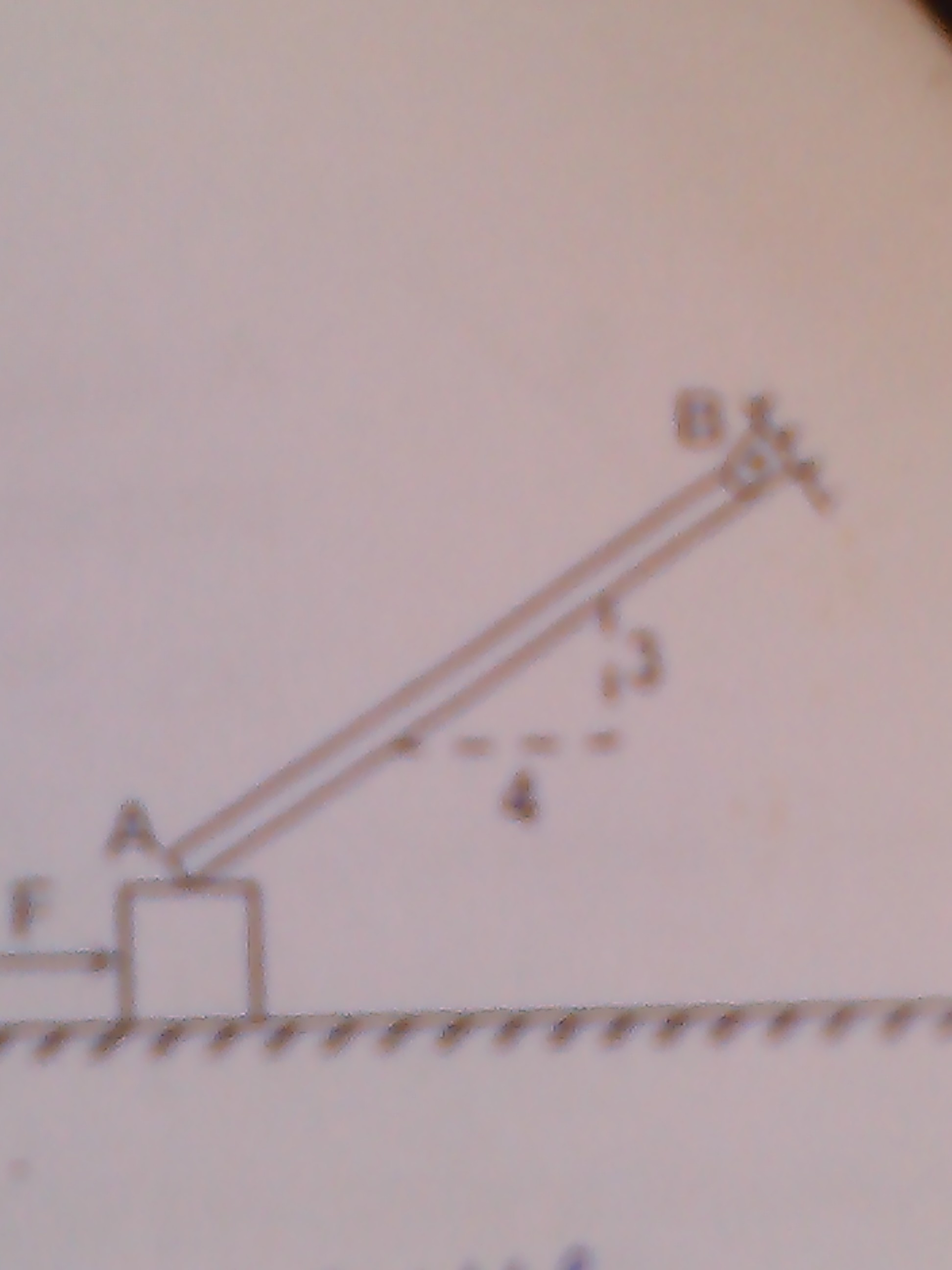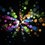# Rotational Dynamics Problem!A uniform bar AB 10 m long and weighing 280 N is hinged at B and rests upon a 400 N block as shown. If the coefficient of friction at all contact surfaces is 0.4. The value of horizontal force F required to start the motion of the 400 N block is...?

For a better image(not much better): http://bit.ly/18yUu8DNote by Siddharth Iyer
6 years, 11 months ago

This discussion board is a place to discuss our Daily Challenges and the math and science related to those challenges. Explanations are more than just a solution — they should explain the steps and thinking strategies that you used to obtain the solution. Comments should further the discussion of math and science.

When posting on Brilliant:

• Use the emojis to react to an explanation, whether you're congratulating a job well done , or just really confused .
• Ask specific questions about the challenge or the steps in somebody's explanation. Well-posed questions can add a lot to the discussion, but posting "I don't understand!" doesn't help anyone.
• Try to contribute something new to the discussion, whether it is an extension, generalization or other idea related to the challenge.

MarkdownAppears as
*italics* or _italics_ italics
**bold** or __bold__ bold
- bulleted- list
• bulleted
• list
1. numbered2. list
1. numbered
2. list
Note: you must add a full line of space before and after lists for them to show up correctly
paragraph 1paragraph 2

paragraph 1

paragraph 2

[example link](https://brilliant.org)example link
> This is a quote
This is a quote
    # I indented these lines
# 4 spaces, and now they show
# up as a code block.

print "hello world"
# I indented these lines
# 4 spaces, and now they show
# up as a code block.

print "hello world"
MathAppears as
Remember to wrap math in $$ ... $$ or $ ... $ to ensure proper formatting.
2 \times 3 $2 \times 3$
2^{34} $2^{34}$
a_{i-1} $a_{i-1}$
\frac{2}{3} $\frac{2}{3}$
\sqrt{2} $\sqrt{2}$
\sum_{i=1}^3 $\sum_{i=1}^3$
\sin \theta $\sin \theta$
\boxed{123} $\boxed{123}$

Sort by:

272 N .

- 6 years, 11 months ago

I am sorry but the correct answer is 320 N. Could you tell me how you approached the problem???

- 6 years, 11 months ago

Ok i got my mistake , i was not considering torque due to friction on the rod.

Draw proper FBD. Let $N_{1}$ and $N_{2}$ be the normal reactions between rod and block and between ground block respectively. Let $f_{1}$ and $f_{2}$ be corresponding frictions.

At the verge of slipping , $f_{1} = 0.4N_{1} , f_{2} = 0.4N_{2}$

On the rod , we can balance torque apout the hinge.

Anticlockwise torque due to friction and weight = clockwise torque due to normal reaction.

$\Rightarrow \frac{mglsin\theta}{2} + 0.4N_{1}lcos\theta = N_{1}lsin\theta$ ,

$\Rightarrow N_{1} = 200N$

Analysing FBD of block ,

$N_{2} = W_{block} + N_{1} = 600N$

$F \geq f_{1} + f_{2} = 0.4(N_{1} + N_{2})$ = $0.4 \times 800 = \fbox{320N}$

- 6 years, 11 months ago

Thanks!!! I figured my mistake too. i was not considering torque due to normal reaction.

- 6 years, 11 months ago

But when I solved the torque equation, I got $N_1$ as 300N. Can you check why?

- 6 years, 11 months ago

Sorry , i can't check without your equations.

- 6 years, 11 months ago

No sorry never mind, I got it.

- 6 years, 11 months ago

A uniform bar AB 10 m long and weighing 280 N is hinged at B and rests upon a 400 N block as shown. If the coefficient of friction at all contact surfaces is 0.4. The value of horizontal force F required to start the motion of the 400 N block is...? what is the solution

- 2 years, 7 months ago